# 7.2   Basic Circuit Model

This chapter under major construction.

A circuit is an interconnected network of wires, power connections, and controlled switches that transforms values on input wires to values on output wires. In our two-dimensional geometric representation, wires correspond to line segments drawn in the plane; controlled switches to wires that cross in a particular manner; and circuits to wires drawn within a rectangle. We encapsulate circuits by drawing the rectangle that defines the circuit boundary. Inputs are wires that terminate at the boundary; outputs stick out past the boundary.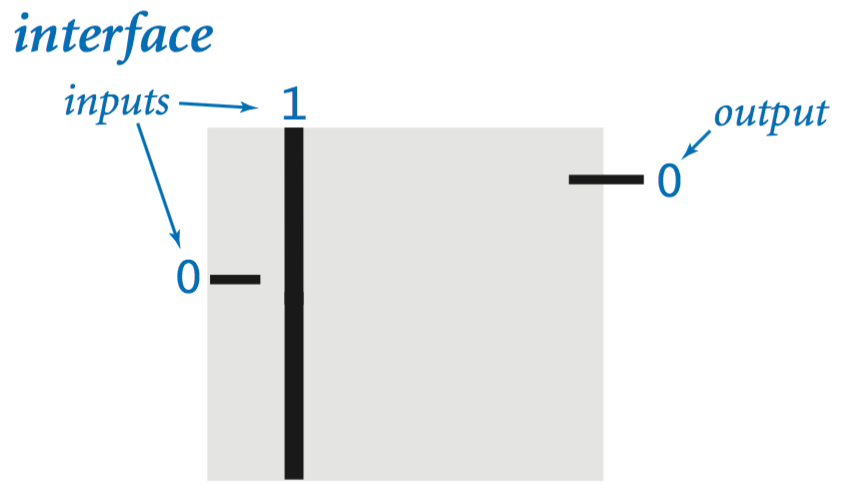## Wires.

Wires connect to power, carry values, and connect to circuit elements. Some of the wires are designated as inputs; some others are designated as outputs. Each wire is always in one of two states (0 or 1). Wires that are connected must have the same value. When we trace the values of wires in a circuit, we represent those with value 1 with thick lines and those with value 0 with thin lines.

## Power sources.

We assume that one of the inputs is always 1 and we use power dots to represent a connection to that input anywhere within a circuit. A wire connected to a power dot has the value 1, unless that connection is broken.

## Controlled switches.

A controlled switch is a place in a circuit where a switch control line crosses another wire and then ends. A change in the value of the switch control line can break the connection to power in the wire it crosses, thereby changing the value of that wire.
• On/off switches. An on/off switch is a wire connected to a power source (1) that is crossed by a switch control line. If the switch control line value is 0, the output wire value is 1; if the control line value is 1, the output wire value is 0.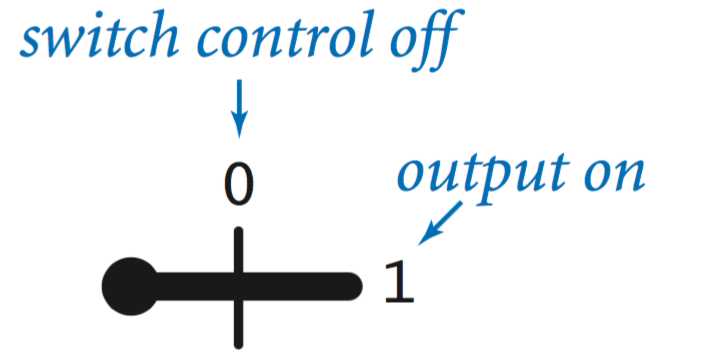• Input/off switches. More generally, we can think of a controlled switch as having an arbitrary input value (not necessarily 1). Logically, the operation of the switch is simple: if the switch control line is 0, then the input and output wires are connected and therefore have the same value (both 0 or both 1); if the switch control line is 1, then the input and output wires are not connected and therefore the value of the output wire is 0 (no matter what the value of the input wire).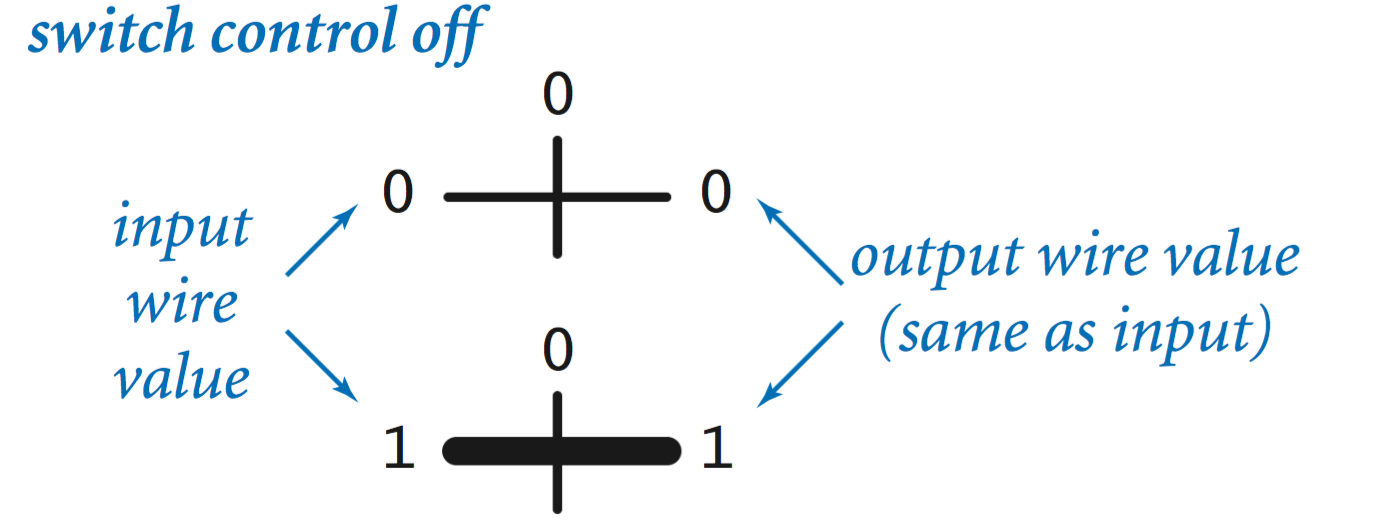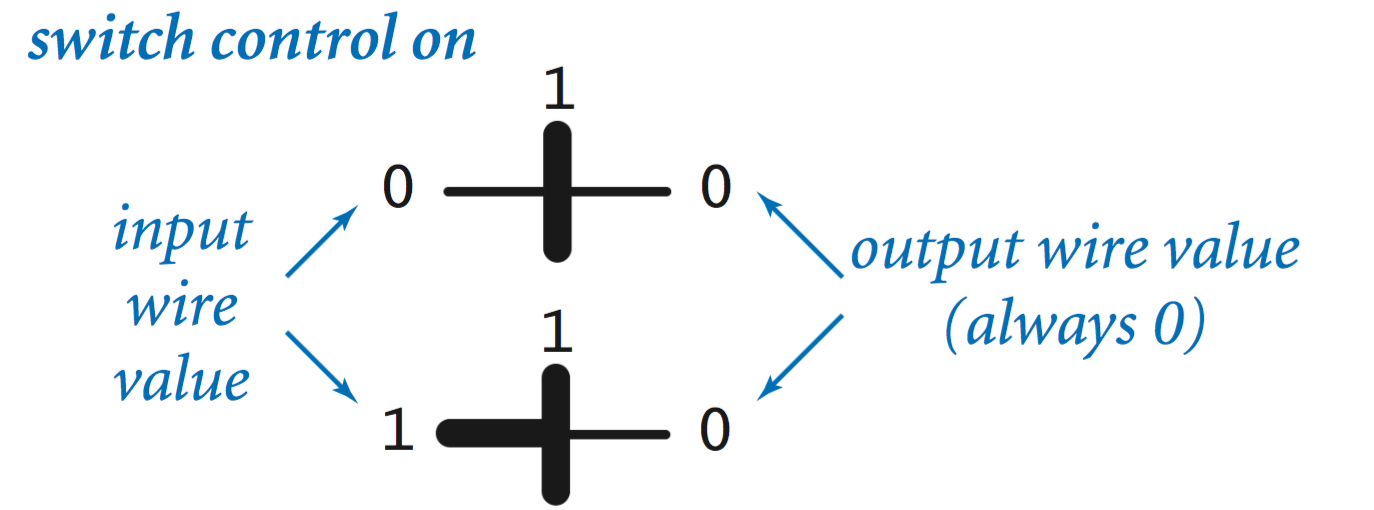• Layout conventions. We do not make an explicit distinction between input and output when drawing controlled switches. That distinction is clear in all of our circuits because inputs are always a wire either connected to a power source or to the output of another switch.
• A physical example. In a relay, the control line is connected to an electromagnet, which attracts a small piece of wire that can connect the input wire to the output wire, but is also connected to a spring. If the electromagnet is off, the spring keeps the input connected to the output; if the electromagnet is on, it exerts a stronger force than the spring to pull the connecting piece so as to break the connection between input and output.## Circuits.

• Switching circuit analysis.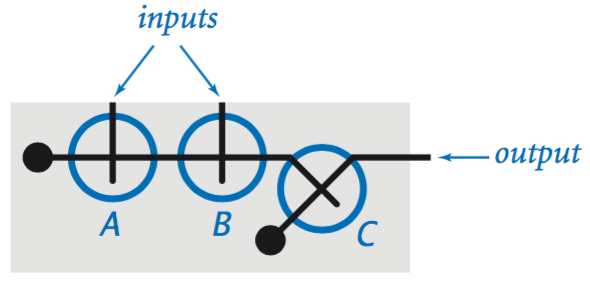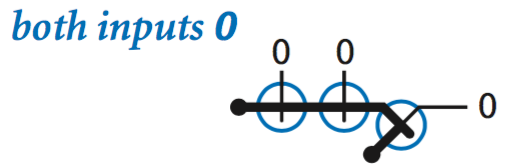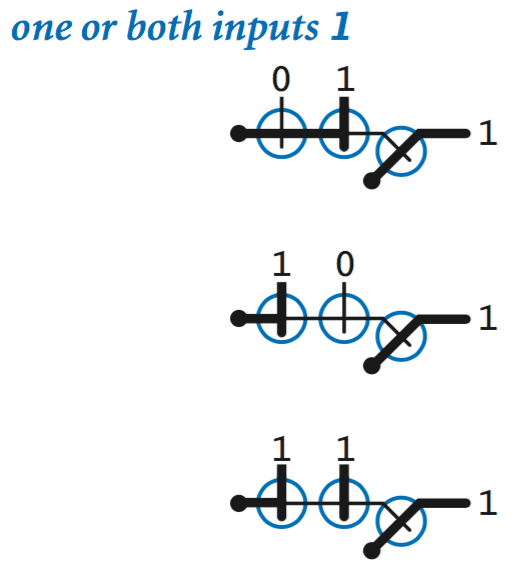• Combinational circuits.
• Sequential circuits.

## Logical design and the real world.

#### Exercises

1. Under which conditions will the output of the following circuit be 0?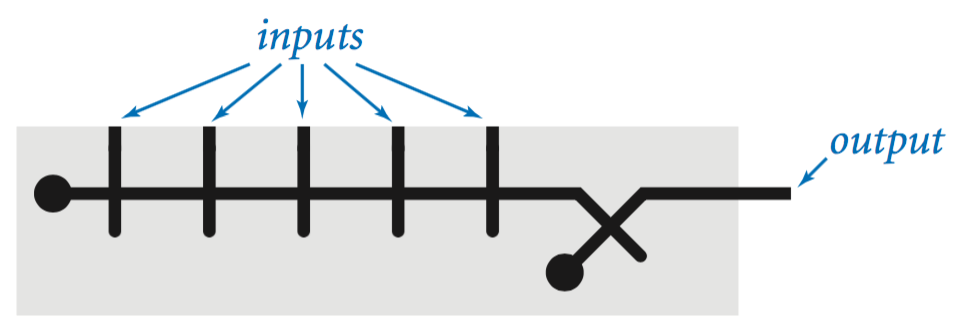Solution: It will be 0 if and only if all of the inputs are 0. It's a multiway NOR gate.

2. State the conditions under which the output of the circuit in the previous exercise will be 1.

Solution: It will be 1 if and only if any of the inputs are 1. It's a multiway OR gate.

3. Under which conditions will the output of the following circuit be 1?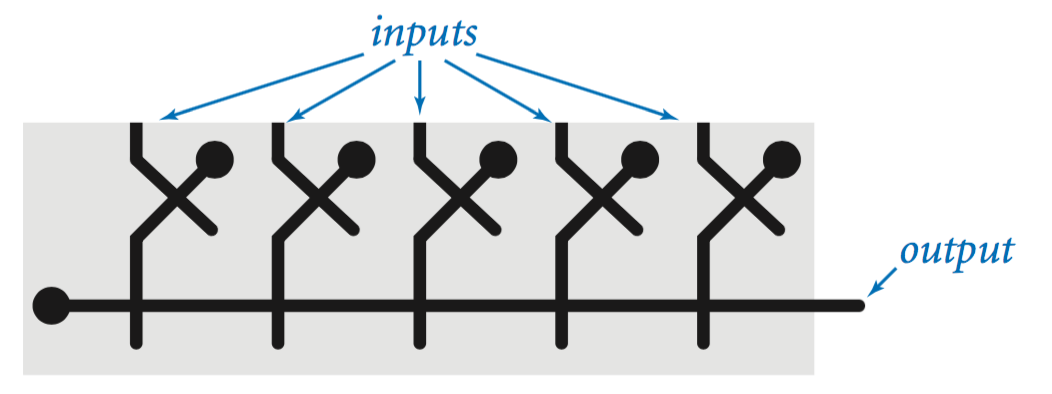Solution: It will be 1 if and only if all of the inputs are 1. It's a multiway AND gate.

4. State the conditions under which the output of the circuit in the previous exercise will be 0.

Solution: It will be 0 if and only if any of the inputs are 0. It's a multiway NAND gate.